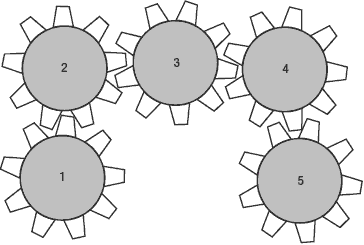# Mechanical Comprehension

15 Questions | Total Attempts: 101SettingsRelated Topics
• 1.
A valve is used to perform which of the following functions?
• A.

Increase the temperature of a gas

• B.

Aid evaporation of a liquid

• C.

Control the flow of a liquid

• D.

Decrease the density of a gas

• 2.
Which term below describes the opposite of an increase in speed?
• A.

Velocity

• B.

Deceleration

• C.

Acceleration

• D.

Rotation

• 3.
Which principle of mechanical motion is used in the design of a roller coaster?
• A.

Momentum

• B.

Friction

• C.

Acceleration

• D.

All of the above

• 4.
A block of steel has a density of 0.29 pounds per cubic inch.  If the block has dimensions of 1 inch by 1 inch by 3 inches, what is the weight?
• A.

0.29 pounds

• B.

0.36 pounds

• C.

0.87 pounds

• D.

0.99 pounds

• 5.
Which mechanical components are typically used between a wheel and an axle to reduce friction?
• A.

Springs

• B.

Bearings

• C.

Hinges

• D.

Levers

• 6.
• A.

Lift objects

• B.

Cut metals

• C.

Cut wood

• D.

Crunch metal

• 7.
• A.

Pulley

• B.

Hoist

• C.

Wheel and axle

• D.

Lever

• 8.
• A.

Contract

• B.

Expand

• C.

Bend

• D.

Harden

• 9.
If a train moves at 70 MPH and travels for 90 minutes, how many miles does it travel?
• A.

105 miles

• B.

105 miles

• C.

109 miles

• D.

630 miles

• 10.
Water is flowing into a tank through pipe A at 6 gallons per minute and  through pipe B at 7 gallons per minute.  The water is flowing out of the tank through pipe C at 8 gallons per minute.  How much more water will be in the tank at the end of 5 minutes.
• A.

15 gallons

• B.

25 gallons

• C.

32 gallons

• D.

50 gallons

• 11.
To lift a 600 pound piano, Tom and Jerry decide to use a plank placed under one end of the piano.  The plank is 3 feet long.  If a fulcrum is placed under the plank, one foot away from the piano, how much force must be applied to the plank to lift the piano?
• A.

100 pounds

• B.

125 pounds

• C.

140 pounds

• D.

150 pounds

• 12.
A garden hose is used to siphon the water out of an above-ground swimming pool.  What makes the water flow out of the pool and through the garden hose?
• A.

Weight of the water

• B.

Water pressure

• C.

Air pressure

• D.

Air and water pressure in combination

• 13.
Which action describes a clock pendulum swaying back and forth?
• A.

Gravity

• B.

Periodic motion

• C.

Acceleration

• D.

Velocity

• 14.
A wrench is used to turn a bolt that has 20 threads per inch.  After 15 complete turns of the wrench, the bolt will have moved:
• A.

1/8 inch

• B.

1/4 inch

• C.

3/4 inch

• D.

2/3 inch

• 15.
Water is flowing into a tank at the rate of 180 gallons per minute.  However, the tank has a leak, and water is leaking out at the rate of 2 gallons per second.  How long will it take to add 120 gallons?
• A.

1 minute

• B.

2 minutes

• C.

3 minutes

• D.

4 minutes